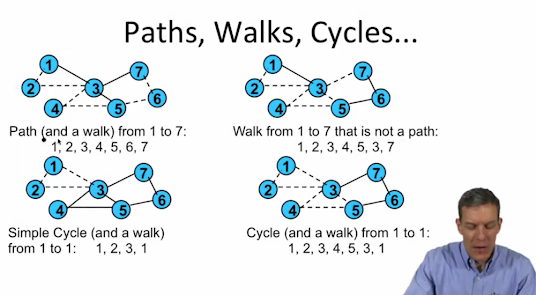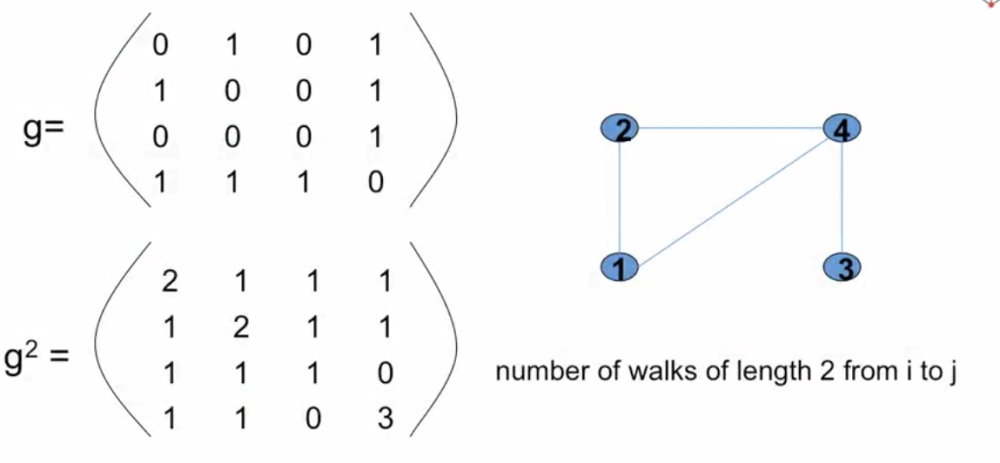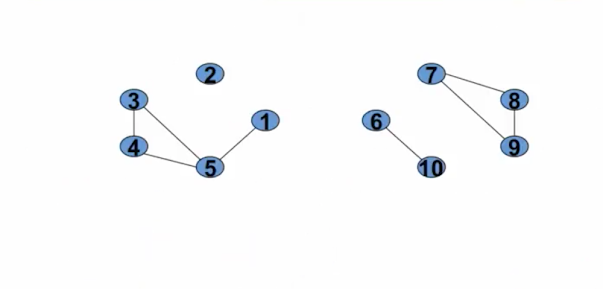# Simplifying the Complexity¶

• Global patterns of networks

• degree distributions, path lengths...
• Segregation Patterns

• node types and homophily
• Local Patterns

• Clustering, Transsitivity, Support...
• Positions in networks

• Neighborhoods, Centrality, Influence...

# Representing Networks¶

• N = {1,...,n} nodes, vertices, players

• g in $\{0,1\}^{n*n}$ adjacency matrix (unweighted, possibly directed)

• $g_{ij} = 1$ indicates a link, tie, or edge between i and j

• Alternative notation: ij in g a link between i and j

• Network(N, g)

# Basic Definitions¶

• Walk from $i_1$ to $i_k$: a sequence of nodes $(i_1, i_2, ..., i_k)$ and sequence of links $(i_1i_2, i_2i_3,...i_{k-1}i_k)$ such that $i_{k-1}i_k$ in g for each k

• Convenient to represent it as the corresponding sequence of nodes $(i_1, i_2, ..., i_k)$ such that $i_{k-1}i_k$ in g for each k

• Path: a walk $(i_1, i_2, ..., i_k)$ with each node $i_k$ distinct

• Cycle: a walk where $i_1 = i_k$

• Geodesic: a shortest path between two nodes# Counting walks:¶How many number of walks of length 2 from i to j:

$g^2$ actually give us the answer of exactly how many walks there are of length 2 from node, whatever to whatever.

Here it says that there's 2 different walks of going from node 1 to node 1:

• go up to node 2 and then back
• go to node 4 and back

So it's impossible to get from node 3 to node 4 in a walk of length 2

And $g^3$ give the number of walks of length 3 from i to j

# Components¶

• (N, g) is connected if there is a path between every two nodes

• Component: maximal connected subgraph

• (N', g') is subset of (N, g)
• (N', g') is connected
• i in N' and ij in g implies j in N' and ij in g'

# A network with four components¶